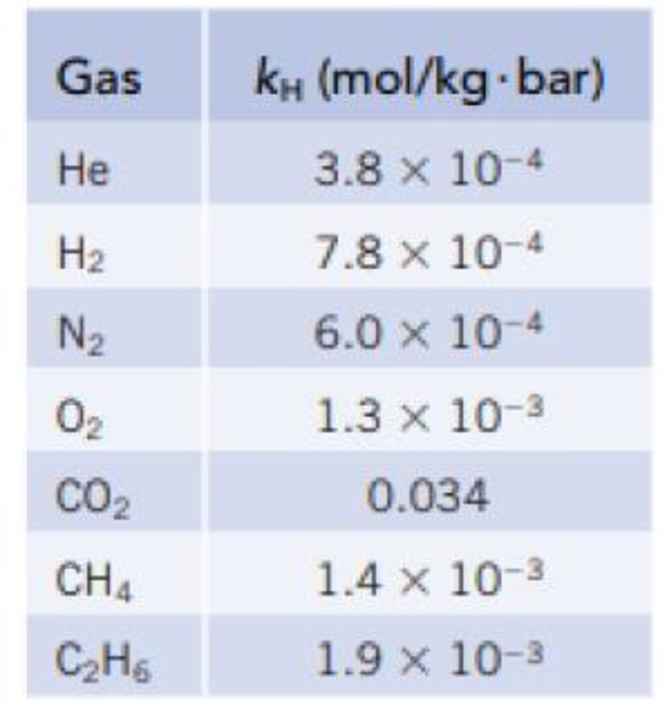Chapter 13, Problem 20PS

Chapter
Section
Textbook Problem

The Henry’s law constant for O2 in water at 25 ° is given in Table 13.2. Which of the following is a reasonable constant when the temperature is 50 °C? Explain the reason for your choice. (a) 6.7 × 10−4 mol/kg · bar (b) 2.6 × 10−3 mol/kg · bar (c) 1.3 × 10−3 mol/kg · bar (d) 6.4 × 10−3 mol/kg · bar TABLE 13.2Henry’s Law Constants for Gases in Water (25 °C)*Interpretation Introduction

Interpretation: The henry constant of oxygen at 50oC to be identified.

Concept introduction:

Henry’s law: The solubility of a gas in liquid is directly proportional to the gas pressure.

Sg= kHPg,where,Sgisthegassolubility(inmol/kg)kHis Henry's constant Pg is the partial pressure of the gaseous solute.

Explanation

Reason for correct answer:

Given:Henry's law constant of O2 at 25oC(KH) 1.3×10-3(mol/Kg.bar)Mole of O2in1Kgof waterat1-bar is 1.3×10-3.1L of water hasmass=1kgConcentration=kH× PO2(Henry's law)1

Still sussing out bartleby?

Check out a sample textbook solution.

See a sample solution

The Solution to Your Study Problems

Bartleby provides explanations to thousands of textbook problems written by our experts, many with advanced degrees!

Get Started

Name the following compounds: SO3 HNO2 Mg3N3 HI(aq) Cu3(PO4)2 CuSO45H2O

General Chemistry - Standalone book (MindTap Course List)

22-83 Which diseases are associated with amyloid plaques?

Introduction to General, Organic and Biochemistry

Why are buffers important for living organisms?

Biology: The Dynamic Science (MindTap Course List)